# JP4161628B2 - Echo suppression method and apparatus - Google Patents

## Info

Publication number
JP4161628B2
JP4161628B2 JP2002210915A JP2002210915A JP4161628B2 JP 4161628 B2 JP4161628 B2 JP 4161628B2 JP 2002210915 A JP2002210915 A JP 2002210915A JP 2002210915 A JP2002210915 A JP 2002210915A JP 4161628 B2 JP4161628 B2 JP 4161628B2
Authority
JP
Japan
Prior art keywords
signal
echo
amount
smoothing
output signal
Prior art date
Legal status (The legal status is an assumption and is not a legal conclusion. Google has not performed a legal analysis and makes no representation as to the accuracy of the status listed.)
Active
Application number
JP2002210915A
Other languages
Japanese (ja)
Other versions
JP2004056453A (en
Inventor

Original Assignee

Priority date (The priority date is an assumption and is not a legal conclusion. Google has not performed a legal analysis and makes no representation as to the accuracy of the date listed.)
Filing date
Publication date
Application filed by 日本電気株式会社 filed Critical 日本電気株式会社
Priority to JP2002210915A priority Critical patent/JP4161628B2/en
Publication of JP2004056453A publication Critical patent/JP2004056453A/en
Application granted granted Critical
Publication of JP4161628B2 publication Critical patent/JP4161628B2/en
Active legal-status Critical Current
Anticipated expiration legal-status Critical

• 238000002592 echocardiography Methods 0.000 title claims description 301
• 230000001629 suppression Effects 0.000 title claims description 77
• 238000009499 grossing Methods 0.000 claims description 118
• 230000000875 corresponding Effects 0.000 claims description 47
• 238000006243 chemical reactions Methods 0.000 claims description 28
• 230000001808 coupling Effects 0.000 claims description 18
• 238000010168 coupling process Methods 0.000 claims description 18
• 238000005859 coupling reactions Methods 0.000 claims description 18
• 230000002194 synthesizing Effects 0.000 claims description 17
• 230000015572 biosynthetic process Effects 0.000 claims description 13
• 238000003786 synthesis reactions Methods 0.000 claims description 13
• 238000004458 analytical methods Methods 0.000 claims description 8
• 230000003247 decreasing Effects 0.000 claims description 4
• 230000001276 controlling effects Effects 0.000 claims description 3
• 239000000428 dust Substances 0.000 claims description 2
• 230000000754 repressing Effects 0.000 claims 1
• 238000004904 shortening Methods 0.000 claims 1
• 238000010586 diagrams Methods 0.000 description 44
• 238000001228 spectrum Methods 0.000 description 37
• 230000003595 spectral Effects 0.000 description 35
• 230000003044 adaptive Effects 0.000 description 21
• 230000000694 effects Effects 0.000 description 13
• 238000000034 methods Methods 0.000 description 7
• 230000001131 transforming Effects 0.000 description 7
• 241001442055 Vipera berus Species 0.000 description 4
• 229920005549 butyl rubber Polymers 0.000 description 3
• 230000002123 temporal effects Effects 0.000 description 3
• 230000001934 delay Effects 0.000 description 2
• 230000000630 rising Effects 0.000 description 2
• 280000606094 Magazine companies 0.000 description 1
• 230000005540 biological transmission Effects 0.000 description 1
• 230000001413 cellular Effects 0.000 description 1
• 230000003111 delayed Effects 0.000 description 1
• 238000001914 filtration Methods 0.000 description 1
• 230000004048 modification Effects 0.000 description 1
• 238000006011 modification reactions Methods 0.000 description 1
• 230000005236 sound signal Effects 0.000 description 1

## Images

•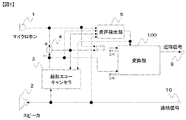•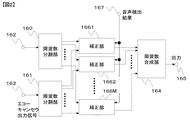•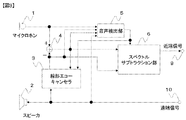•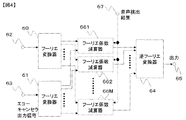••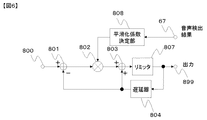•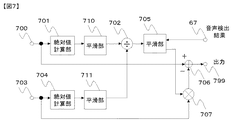•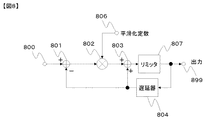•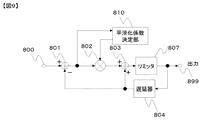•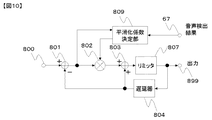•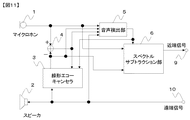•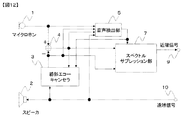•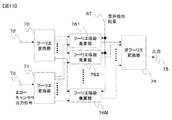•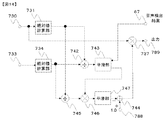••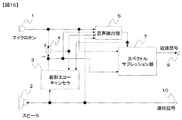•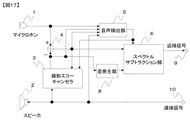•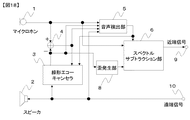•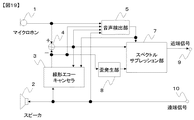•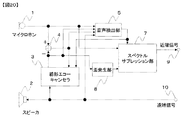•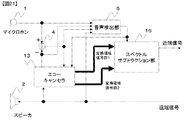•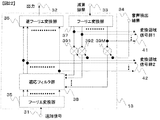•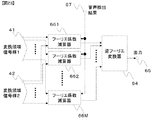•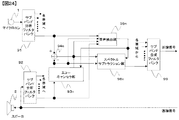•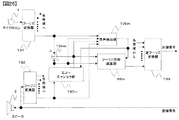••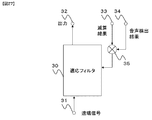## Classifications

• HELECTRICITY
• H04ELECTRIC COMMUNICATION TECHNIQUE
• H04MTELEPHONIC COMMUNICATION
• H04M9/00Interconnection arrangements not involving centralised switching
• H04M9/08Two-way loud-speaking telephone systems with means for suppressing echoes or otherwise conditioning for one or other direction of traffic
• H04M9/082Two-way loud-speaking telephone systems with means for suppressing echoes or otherwise conditioning for one or other direction of traffic using echo cancellers

## Description


BACKGROUND OF THE INVENTION
The present invention relates to an echo suppression technique in a situation where loudspeaking and sound collection are performed at the same time, such as a hands-free telephone and a voice recognition device while reproducing a car stereo.

[Prior art]
FIG. 26 shows a configuration example of the prior art that suppresses echoes due to acoustic coupling between the sound collector and the loudspeaker in the hands-free telephone. In FIG. 26, the voice signal (referred to as a far-end signal) of the other party to be applied to the input terminal 10 is amplified as far-end voice from the speaker 2. A necessary sound, for example, a voice of a speaker (referred to as a near-end voice) enters the microphone 1, and a far-end voice amplified by the speaker 2 leaks through the space. This leakage is called echo. An acoustic transmission system including the speaker 2 and the microphone 1 from the far-end signal to the output signal of the microphone 1 is called an echo path.

It is only the near-end sound that is desired to be sent as a near-end signal from the output terminal 9, and it is desired to remove the echo of the far-end sound that has been loudly leaked from the speaker 2. If the far-end speech leaks greatly, the far-end speech that has been delayed will be heard as an echo on the other side, making it difficult to speak at the far end. Conventionally, a technique that is generally applied to this problem is a technique that uses a linear echo canceller. The linear echo canceller is described, for example, in a paper “The hands-free telephone problem: an annotated bibliography update” by Eberhard HANSLER (Source: annars of telecommunications, 1994, p360-367) (hereinafter referred to as reference 1).

In FIG. 26, the linear echo canceller 3 simulates the transfer function of the echo path. Using this simulated transfer function, a signal simulating an echo leaking into the sound received by the microphone 1, that is, an echo replica signal, is generated from an input signal (far-end signal) to the speaker 2. A near end signal is generated by subtracting from the received sound signal of 1. The voice detector 5 detects whether there is near-end voice by inputting the output of the microphone 1, the output of the linear echo canceller 3, the output of the subtractor 4, and the far-end signal, respectively. When there is sound, 0 or an extremely small value is output as the sound detection result, and when there is no near-end sound, a large value is output.

The operation of the linear echo canceller 3 will be described with reference to FIG. FIG. 27 is a diagram illustrating a configuration example of the linear echo canceller 3. The configuration example of the linear echo canceller illustrated in FIG. 27 includes an adaptive filter 30 and a multiplier 35. The adaptive filter 30 receives the far-end signal input from the terminal 31 and outputs the result of the linear filter from the terminal 32. Here, the filter coefficient for performing the linear filter operation in the adaptive filter 30 is updated every moment. The update is performed using a correlation operation so as to minimize the subtraction result received from the terminal 33. As a result, in the subtraction result applied to the terminal 33, the component having a correlation with the far-end signal is minimized. That is, the far-end signal echo is removed.

The multiplier 35 is inserted in the path from the terminal 33 to the adaptive filter 30 in order to control the amount of filter coefficient update in the adaptive filter 30. If there is no multiplier 35 and the filter coefficient is updated in the adaptive filter 30 when there is near-end speech, the filter coefficient is temporarily disturbed, and the amount of echo removal is reduced. The multiplier 35 sends the result obtained by multiplying the subtraction result received from the terminal 33 by the voice detection result from the voice detection unit 5 received from the terminal 34 to the adaptive filter 30. When there is near-end speech, the speech detection result is 0 or an extremely small value, so that update of the filter coefficient in the adaptive filter 30 is suppressed, and the filter coefficient is not disturbed. As a result, a high echo removal amount can be obtained.

As described above, the conventional linear echo canceller can remove the echo of the far-end signal using the linear adaptive filter. Various configurations such as an FIR type, an IIR type, and a lattice type can be used for the adaptive filter.

[Problems to be solved by the invention]
Also in the prior art, when nonlinear elements such as distortion are sufficiently small in the echo path, the echo can be sufficiently suppressed. However, in an actual device, nonlinear elements such as distortion in a speaker are large. The transfer function of the echo path including distortion is non-linear and cannot be completely simulated by a linear echo canceller. In particular, when a loud speaker is used with a small speaker used in a cellular phone or the like, since the distortion of the speaker is large, the echo is suppressed only by about 20 dB. In this case, since the echo is sent to the far end as a near end signal and can be heard by the far end speaker, it becomes difficult to speak.

An object of the present invention is to perform sound collection in which echo is sufficiently suppressed even when distortion in the echo path is large.

[Means for Solving the Problems]
A first echo suppression method of the present invention is a method for suppressing echoes due to acoustic coupling between a sound collector and a loudspeaker. In this method, the output of the echo canceller is output from the output signal of the sound collector or the output signal of the sound collector. When any one of the subtracted signals is the first signal and the output signal of the echo canceller is the second signal, the echo leakage is estimated from the first signal and the second signal. A value is calculated, and the first signal is corrected based on the calculated estimated value. In addition, as the estimated value, an amount corresponding to the amplitude or power of the first signal with respect to an amount obtained by smoothing the amount corresponding to the amplitude or power of the second signal in a period in which no near-end speech is detected. Use the smoothed value for the ratio of the smoothed amount .

According to a second echo suppression method of the present invention, in the first echo suppression method, echo leakage from the first signal and the second signal is performed for each frequency component of the first and second signals. An estimation value of the degree of dust is calculated, and a process of correcting the first signal based on the calculated estimation value is performed.

According to a third echo suppression method of the present invention, in the second echo suppression method, instead of using the output signal of the echo canceller as the second signal, a signal in which distortion of the output signal of the echo canceller is emphasized is used. The second signal is assumed.

The first echo suppressor of the present invention is an apparatus that suppresses echo due to acoustic coupling between a sound collector and a loudspeaker, and outputs an echo canceller from an output signal of the sound collector or an output signal of the sound collector. When any one of the subtracted signals is the first signal and the output signal of the echo canceller is the second signal, the echo leakage is estimated from the first signal and the second signal. A conversion unit including a calculation unit that calculates a value and a correction unit that corrects the first signal based on the calculated estimated value; And the calculation means uses the amplitude or power of the first signal relative to an amount obtained by smoothing an amount corresponding to the amplitude or power of the second signal in a period in which near-end speech is not detected as the estimated value. Use the smoothed value for the ratio of the smoothed amount corresponding to .

The second echo suppressor of the present invention is an apparatus that suppresses echoes due to acoustic coupling between the sound collector and the loudspeaker, and outputs the echo canceller from the output signal of the sound collector or the output signal of the sound collector. A dividing means for frequency-dividing the first and second signals when any one of the subtracted signals is a first signal and the output signal of the echo canceller is a second signal; Calculation means for calculating an estimated value of echo leakage from the first signal and the second signal for each frequency component of the second signal, and based on the estimated value calculated for each frequency component And a conversion unit including correction means for correcting the first signal for each frequency component and combining means for combining the first signal corrected for each frequency component. And the calculation means uses the amplitude or power of the first signal relative to an amount obtained by smoothing an amount corresponding to the amplitude or power of the second signal in a period in which near-end speech is not detected as the estimated value. Use the smoothed value for the ratio of the smoothed amount corresponding to .

The third echo suppressor of the present invention emphasizes the distortion of the output signal of the echo canceller by the distortion generator instead of using the output signal of the echo canceller as the second signal in the second echo suppressor. Let the signal be a second signal.

A fourth echo suppression apparatus of the present invention is an apparatus that suppresses an echo caused by acoustic coupling between a sound collector and a loudspeaker, and is an echo canceller. A signal simulating an echo leaking from the input signal of the loudspeaker into the received sound of the sound collector for each frequency component of the input signal of the loudspeaker and the signal obtained by subtracting the output signal of the echo canceller from the output signal of the sound collector Generate components and synthesize the signal components generated for each frequency component to generate the output signal of the echo canceller A signal having a conversion domain echo canceller, which is obtained by subtracting the output signal of the conversion domain echo canceller from the output signal of the sound collector Each frequency component of A first signal, The signal component generated for each frequency component is Calculating means for inputting an estimated value of echo leakage from the first signal and the second signal for each frequency component of the first and second signals, respectively. A correcting means for correcting the first signal for each frequency component based on an estimated value calculated for each frequency component; and a combining means for combining the first signal corrected for each frequency component. Has a conversion part including And the calculation means uses the amplitude or power of the first signal relative to an amount obtained by smoothing an amount corresponding to the amplitude or power of the second signal in a period in which near-end speech is not detected as the estimated value. Use the smoothed value for the ratio of the smoothed amount corresponding to .

A fifth echo suppressor of the present invention is a device for suppressing echoes due to acoustic coupling between a sound collector and a loudspeaker, wherein the output signal of the sound collector and the output signal of the loudspeaker are subband analysis filters. 1 is obtained by subtracting the output signal of the sound collector developed in each band or the output signal of the echo canceller from the output signal of the sound collector developed in each band. A calculation means for calculating an estimated value of echo leakage from the first signal and the second signal when the signal and the output signal of the echo canceller are the second signal, and the calculated estimated value And a sub-band synthesis filter for synthesizing the first signal corrected by each conversion unit. In addition, the calculation means uses, as the estimated value, the amplitude or power of the first signal with respect to an amount obtained by smoothing an amount corresponding to the amplitude or power of the second signal in a period in which near-end speech is not detected. Use the smoothed value for the ratio of the smoothed amount corresponding to .

A sixth echo suppressor of the present invention is an apparatus for suppressing echoes due to acoustic coupling between a sound collector and a loudspeaker, and the output signal of the sound collector and the output signal of the loudspeaker are converted by a Fourier transformer. Either the output signal of the sound collector developed in each band, or the signal obtained by subtracting the output signal of the echo canceller from the output signal of the sound collector developed in each band is the first signal. When the output signal of the echo canceller is the second signal, a calculation means for calculating an estimated value of echo leakage from the first signal and the second signal, and a calculated estimated value And a conversion unit including a correction unit that corrects the first signal based on each band, and an inverse Fourier transformer that synthesizes the first signal corrected by each conversion unit. In addition, the calculation means uses, as the estimated value, the amplitude or power of the first signal with respect to an amount obtained by smoothing an amount corresponding to the amplitude or power of the second signal in a period in which near-end speech is not detected. Use the smoothed value for the ratio of the smoothed amount corresponding to .

The smoothing time constant of the amount corresponding to the amplitude or power of the first and second signals is controlled to be smaller when the first and second signals increase than when the first and second signals decrease. You can do it. Further, the time constant for smoothing the ratio may be controlled to be long or infinite when near-end speech is detected, and to be shortened otherwise, and no near-end speech is detected. It is controlled so that it is much larger when it is detected, and when the near-end speech is not detected, it is smaller when the ratio increases than when it decreases. May be.

The correction may be performed by estimating the amount of echo included in the first signal from the estimated value and the second signal, and subtracting the estimated amount of echo from the first signal. It is good to estimate the ratio of the near-end signal included in the first signal from the estimated value and the first and second signals, and multiply the first signal by the estimated ratio. Also good.

[Action]
If the echo canceller is a linear echo canceller, the harmonic component contained in the far-end signal appears almost as it is in the output of the echo canceller. Moreover, even if this echo canceller is a nonlinear echo canceller, not only a few harmonic components are included in the far-end signal. On the other hand, the near-end signal that is the input signal of the sound collector includes not only the near-end speech but also harmonics generated by the echo and nonlinear components of the far-end signal due to acoustic coupling between the sound collector and the loudspeaker. In the state where the component is included but there is no near-end speech, the near-end signal is almost only the harmonic component generated by the echo and the nonlinear component of the far-end signal. For this reason, the amount corresponding to the amplitude or power of the first signal (near-end signal itself or the output of the echo canceller is subtracted from the near-end signal) in a state where there is no near-end sound is set to the second signal (echo The quotient divided by the amount corresponding to the amplitude or power of the canceler output signal itself or the signal with enhanced distortion) is an estimate of the gain of the nonlinear component of the echo in the echo path, that is, an estimate of the echo leakage. Become. Accordingly, the amount of echo contained in the first signal is estimated from the estimated value and the second signal and subtracted from the first signal, or from the estimated value and the first and second signals. By estimating the ratio of the near-end signal included in the first signal and multiplying the first signal by this estimated ratio, the nonlinear component of the echo can be removed from the first signal.

DETAILED DESCRIPTION OF THE INVENTION
Next, embodiments of the present invention will be described with reference to the drawings.

Referring to FIG. 1, an embodiment of the present invention uses a linear echo canceller (or a linear echo canceller) from an output signal of a microphone 1 or an output signal of a speaker 2 in order to suppress an echo due to acoustic coupling between the microphone 1 and the speaker 2. Any one of the signals obtained by subtracting the output signal 3 by the subtractor 4 may be the first signal, the output signal of the linear echo canceller 3, or the distortion included in the output signal (not shown). The near-end signal obtained by inputting the signal emphasized by the generation unit as the second signal and the voice detection signal of the voice detection unit 5 that detects the presence or absence of the near-end voice and removing the echo from the first signal. Is generated and output to the output terminal 9. Here, the conversion unit 100 calculates an estimated value of echo leakage from the first signal and the second signal, and corrects the first signal based on the calculated estimated value. This process is preferably performed for each frequency component of the first and second signals.

Referring to FIG. 2, the conversion unit 100 includes a frequency division unit 160 that divides the first signal applied from the input terminal 162 into M for each frequency, and a frequency division unit that divides the second signal applied from the input terminal 163 into M for each frequency. 161, M correction units 166m (m = 1 to M) provided for each frequency, and a frequency synthesis unit 164. The frequency division unit 160 and the frequency division unit 161 each include a first signal obtained by frequency division and The second signal is sent to the correction unit 166m corresponding to each frequency, and each correction unit 166m receives the voice detection result of the voice detection unit 5 input from the input terminal 167, the first signal in the frequency component, and the second signal. An estimated value of echo leakage is calculated from the above signal, and the first signal is corrected based on this estimated value and output to the frequency synthesizer 164. The frequency synthesizer 1 4 outputs the signal output from the correction unit 166m in frequency synthesis to the output terminal 165.

Here, the correction unit 166m uses the amplitude or power of the first signal with respect to the amount corresponding to the amplitude or power of the second signal in the period in which the near-end speech is not detected as the estimated value of the echo leakage. A ratio of quantities or a value obtained by smoothing this ratio is used. An amount corresponding to the amplitude or power of the first and second signals may be smoothed. The smoothing time constant of the amount corresponding to the amplitude or power of the first and second signals is controlled to be smaller when the first and second signals increase than when the first and second signals decrease. You can do it. The time constant for smoothing the ratio may be long or infinite when near-end speech is detected, and may be shortened otherwise, compared to when no near-end speech is detected. It is also possible to make the direction when it is detected much larger, and when the near-end speech is not detected, the time when the ratio increases is smaller than when the ratio decreases.

The correction unit 166m estimates the amount of echo included in the first signal from the estimated value of the echo leakage and the second signal, and subtracts the estimated amount of echo from the first signal. Therefore, the first signal may be corrected, or the ratio of the near-end signal included in the first signal is estimated from the estimated value of the echo leakage and the first and second signals. The first signal may be corrected by multiplying the first signal by the estimated ratio.

The frequency division in the frequency division units 160 and 161 can use any linear transformation such as Fourier transform, cosine transformation, and subband analysis filter bank, and the frequency synthesis in the frequency synthesis unit 164 is the inverse Fourier transform corresponding to them. Any processing such as inverse cosine transform, subband synthesis filter bank, etc. can be used.

Next, embodiments of the present invention will be described with reference to the drawings. First, an embodiment using a spectrum subtraction unit as the conversion unit 100 of FIG. 1 will be described.

[First embodiment]
FIG. 3 is a block diagram of the first embodiment of the present invention. 26 differs from the prior art in FIG. 26 in that a spectral subtraction unit 6 is inserted between the subtracter 4 and the near-end signal output terminal 9 in FIG. It is that the output signal of the canceller 3 is received and the sound detection result output from the sound detection unit 5 is received.

The spectral subtraction unit 6 develops the output signal of the subtractor 4 and the output signal of the linear echo canceller 3 in the frequency domain, and removes the echo for each frequency. The configuration example and operation will be described with reference to FIG.

FIG. 4 is a block diagram illustrating a configuration example of the spectrum subtraction unit 6. The output signal of the subtracter 4 in FIG. 3 is input from the input terminal 62. The Fourier transformer 60 receives the signal input from the input terminal 62, calculates its M-point Fourier transform, and uses the calculated result as the first Fourier coefficient, the Fourier coefficient subtractor 66m (corresponding to each frequency). m = 1 to M). On the other hand, an output signal of the linear echo canceller 3 in FIG. 3, that is, an echo replica signal is input from the input terminal 63. The Fourier transformer 61 receives the signal input from the input terminal 63, calculates its M-point Fourier transform, and uses the calculated result as the second Fourier coefficient to the Fourier coefficient subtractor 66m corresponding to each frequency. send.

In the Fourier coefficient subtractor 66m, the first Fourier coefficient output from the Fourier transformer 60, the second Fourier coefficient output from the Fourier transformer 61, and the voice detection result (terminal) output from the voice detector 5 in FIG. 67), the Fourier coefficient from which the echo component is removed is calculated, and the calculation result is sent to the inverse Fourier transformer 64. The inverse Fourier transformer 64 receives the Fourier coefficient group output from the Fourier coefficient subtraction units 661 to 66M, calculates the inverse Fourier transform, and outputs the real part of the calculation result from the output terminal 65.

Next, each configuration example and operation of the Fourier coefficient subtractor 66m (m = 1 to M) will be described with reference to FIG. FIG. 5 is a block diagram showing a first configuration example of the Fourier coefficient subtractor 66m (m = 1 to M). The first Fourier coefficient for each frequency output from the Fourier transformer 60 in FIG. 4 is sent to the absolute value calculator 701 and the subtractor 706 through the terminal 700. The second Fourier coefficient output from the Fourier transformer 61 in FIG. 4 is sent to the absolute value calculator 704 and the multiplier 707 through the terminal 703. The absolute value calculation unit 701 receives the first Fourier coefficient, calculates the absolute value, and sends the calculation result to the divider 702. The absolute value calculation unit 704 receives the second Fourier coefficient, calculates the absolute value, and sends the calculation result to the divider 702. The divider 702 receives the calculation result of the absolute value calculation unit 701 and the calculation result of the absolute value calculation unit 704, and calculates a value obtained by dividing the calculation result of the absolute value calculation unit 701 by the calculation result of the absolute value calculation unit 704. The calculation result is sent to the smoothing unit 705.

Here, the absolute value of the Fourier coefficient, that is, the absolute value of the amplitude of the frequency component is obtained by the absolute value calculation units 701 and 704, but the square root of the root mean square of the amplitude and the absolute value of the amplitude are also obtained. Any other amount can be used as long as the amount is proportional to the amplitude, such as the Q-th root of the Q-power average (Q is an arbitrary positive real number). Also, an amount proportional to power, for example, an even power such as a square can be used. The same applies to the other embodiments described below.

Smoothing section 705 smoothes the calculation result received from divider 702 and sends the result to multiplier 707. The smoothing time constant in the smoothing unit 705 is controlled by the sound detection result input from the terminal 67. When the voice detection result indicates that there is voice (near-end voice), the smoothing time constant is set to be long or infinite. When the speech detection result indicates that there is no speech (near-end speech), the smoothing time constant is shortened. The configuration of the smoothing unit 705 will be described later.

Multiplier 707 multiplies the value output from smoothing unit 705 by the second Fourier coefficient received from Fourier transformer 61 through terminal 703, and sends the result to subtractor 706. The subtractor 706 subtracts the value output from the multiplier 707 from the first Fourier coefficient received from the Fourier transformer 60 through the terminal 700 and outputs the calculation result from the terminal 799. The calculation result output through the terminal 799 is sent to the inverse Fourier transformer 64 in FIG.


The smoothing unit 705 constitutes a so-called leak integrator or a so-called primary IIR type low-pass filter. However, the coefficient for determining the time constant is not a constant, but is supplied as a time-varying coefficient from the smoothing coefficient determination unit 808. Note that the smoothing coefficient and the smoothing time constant have an inversely proportional relationship.

The smoothing coefficient determination unit 808 supplies different smoothing coefficients depending on the sound detection result supplied from the terminal 67. If there is speech (near-end speech), a very small non-negative coefficient, for example, 0.0 is supplied. When there is no voice (near-end voice), a relatively large positive coefficient, for example, 0.005 is supplied. When there is speech (near-end speech) due to these time-varying smoothing coefficients, the smoothing coefficient one sample before is maintained or the smoothing coefficient hardly changes. Further, when there is no voice (near-end voice), the output smoothing coefficient changes.

By this control, the output of the smoothing unit 705 becomes an estimated value of echo leakage at that frequency. The reason will be described. Since the input of the smoothing unit 705 is a quotient obtained by dividing the Fourier coefficient of the near-end signal by the Fourier coefficient of the far-end signal, the Fourier coefficient of the near-end signal is obtained by multiplying this quotient by the Fourier coefficient of the linear echo canceller output signal. The same value as can be generated. That is, it is a value representing the degree of echo leakage. This value is a value that changes drastically, and when there is a voice (near-end voice), it is greatly disturbed and cannot be trusted as it is. However, smoothing provides a stable and reliable estimate. Further, by controlling the smoothing constant so that the change is slow or does not change when there is a voice (near-end voice), the influence of disturbance when there is a voice (near-end voice) can be reduced. As a result, the output of the smoothing unit 705 is an estimated value that is better when it is controlled by the sound detection result as an estimated value of echo leakage at that frequency than when it is not controlled.

The multiplier 707 multiplies the Fourier coefficient of the linear echo canceller output signal by the value output from the smoothing unit 705 obtained as described above, whereby the estimated value of the Fourier coefficient of the echo signal remaining in the echo replica signal is obtained. can get. By subtracting the estimated value of the Fourier coefficient of the echo signal from the Fourier coefficient of the near-end signal in the subtractor 706, an estimated value of the Fourier coefficient of the near-end signal with the echo component suppressed is obtained. The estimated value is sent to the inverse Fourier transformer 64 in FIG. 4, synthesized into a near-end signal, and output from the output terminal 65. As a result, echo is suppressed in this synthesized near-end signal.

The operation of the above Fourier coefficient subtractor 66m will be described using equations. Let S be the Fourier coefficient of the near-end signal, A of the near-end speech component is E, E is the echo component, and N is the noise component. here,
S = A + E + N (Formula 1)
There is a relationship. Also, let R be the Fourier coefficient of the far-end signal. Since there is a time axis, the phases of E and R should be almost the same. When there is no A, that is, when there is no near-end speech, the near-end signal is E + N, and all signals can be erased. Assume that E + N is estimated from R using the signal in this case, and E + N is subtracted from the near-end signal when there is near-end speech. The result P1 (corresponding to the output of the smoothing unit 705) obtained by smoothing the S / R only when there is no near-end speech using the speech detection result is:
P1 = Av [S / R] = Av [(E + N) / R] (Formula 2)
It becomes. Here, Av [•] represents smoothing. This P1 approximates how much of the far-end signal R leaks as an echo, and can be said to be an echo gain in the echo path.

Therefore, the result P2 (corresponding to the output of the multiplier 707) obtained by multiplying P1 by R is an estimated value of the echo component and the noise component.
P2 = P1 x R
= R × Av [(E + N) / R]
= Ex [E + N] (Formula 3)
Here, Ex [•] represents an estimated value.

The result P3 obtained by subtracting P2 from S (corresponding to the output of the subtractor 706) is:
P3 = S-P2
= S− (R × Av [(E + N) / R])
= (A + E + N) −Ex [E + N]
= Ex [A] (Formula 4)
It becomes. In P3, an estimated value of the Fourier coefficient component A of the near-end speech from which the echo component E and the noise component N are removed is obtained.

Returning to FIG. 3, how the embodiment of the present invention operates when the speaker in the echo path is distorted will be described. In the case where there is distortion, in the embodiment of the present invention, the component due to distortion in the echo is removed by nonlinear calculation in the frequency domain of the spectral subtraction unit 6. The linear echo canceller 3 adjusts the temporal change of the signal component, which is important in the frequency domain nonlinear calculation, thereby effectively removing the component due to distortion in the echo. The output signal of the microphone 1 includes a distortion echo of the far end signal in addition to the far end signal itself. This distortion echo can be considered as a harmonic of the frequency component of the far-end signal echo. To simplify the explanation, consider a case where the echo component E is only a harmonic due to distortion. As can be seen from Equations 2 and 3, the spectral subtraction unit can theoretically remove the echo component E as long as the Fourier transform component R of the far-end signal is not zero. Here, what is important for removing the echo component E is the estimation accuracy of the echo gain P1 in the echo path. Since the amount of harmonics due to distortion varies greatly with time due to the nature of the far-end signal, such as amplitude, in order to obtain high accuracy in P1, the denominator on the right side of Equation 1, that is, the Fourier transform of the far-end signal. It is desirable that the time change of the component R is as similar as possible to the time change of the echo component E in the molecule. If these temporal changes are greatly different, high accuracy cannot be obtained as P1, and distortion echoes cannot be largely removed. In the embodiment of the present invention, since the value based on the output signal of the linear echo canceller 3 is used as R, the timings of temporal changes in E and R are substantially aligned. That is, it is close to a desirable state for obtaining high estimation accuracy in P1. Therefore, the spectral subtraction unit 6 can greatly suppress harmonics generated by distortion.

The first embodiment of the present invention also has an effect of removing residual echoes when the echo path estimation is incorrect in the linear echo canceller 3 of FIG. In the description of the previous paragraph, the case where the echo component E is only a harmonic due to distortion is considered for the sake of simplicity, but the echo component of the far-end signal that does not depend on distortion, that is, an echo component that is not a harmonic exists. The discussion is also the same, and it is possible to suppress echo components that are not harmonics. For example, the estimation of the echo path may be wrong, and the subtracter 4 in FIG. 3 may add the reverse without removing the echo. However, even in that case, since the component of the far-end signal is removed in the spectrum subtraction unit 6, the echo can be suppressed.

Further, when this effect of the two-input spectrum subtraction unit 6 is used, the amount of calculation can be reduced by reducing the number of taps of the linear echo canceller 3. As shown in FIG. 26, when only the linear echo canceller is used, the echo removal amount is reduced by reducing the number of taps. However, in the first embodiment of the present invention shown in FIG. 3, even if the number of taps is reduced, the decrease in the echo removal amount is small and a practical echo removal amount can be obtained.

As described above, in the first embodiment of the present invention, the combination of the linear echo canceller 3 and the nonlinear calculation in the frequency domain of the spectrum subtraction unit 6 compensates for weak points and improves performance. It has gained. That is, even if the echo cannot be sufficiently suppressed by the linear echo canceller 3 alone, such as when there is distortion in the echo path or when the linear echo canceller 3 erroneously estimates the echo path due to the spectral subtraction unit 6, It can be greatly suppressed. In addition, by using the output of the linear echo canceller, it is possible to suppress harmonics caused by distortion by simple estimation of only the amplitude without worrying about the time lag that cannot be handled by the frequency domain calculation of the spectral subtraction unit. it can.

FIG. 7 is a second configuration example of the Fourier coefficient subtractor 66m (m = 1 to M) in the spectral subtraction unit 6 of FIG. The difference from the first configuration example of the Fourier coefficient subtracter shown in FIG. 5 is that the smoothing unit 710 is inserted in the signal path from the absolute value calculation unit 701 to the divider 702 and the absolute value calculation. The only difference is that a smoothing unit 711 is inserted in the signal path from the unit 704 to the divider 702.

A configuration example of the smoothing unit 710 and the smoothing unit 711 is shown in FIG. FIG. 8 is a so-called leak integrator or first-order IIR type low-pass filter. Since the operation is very similar to the configuration example of the smoothing unit 705 shown in FIG. 6, the operation will be described while comparing FIG. 8 with FIG. The only difference between FIG. 8 and FIG. 6 is that the smoothing coefficient supplied to the multiplier 802 is supplied as a smoothing constant at the terminal 806. In the configuration example of FIG. 6, the input signal is smoothed without being affected by the voice detection result, whereas the configuration example of FIG. 8 is influenced by the voice detection result.

In the second configuration example of the Fourier coefficient subtractor 66m (m = 1 to M) illustrated in FIG. 7, the smoothing unit 710 and the smoothing unit 711 smooth the two inputs of the divider 702. The quotient input to the smoothing unit 705 as the output of the device 702 is also smoothed. As a result, the estimated value that is the output of the smoothing unit 705 is more stable in the second configuration example in FIG. 7 than in the first configuration example in FIG. However, in both the first configuration example and the second configuration example, the function of obtaining an estimated value of echo leakage at the output of the smoothing unit 705 remains the same. Therefore, even when the second configuration example shown in FIG. 7 is used as the Fourier coefficient subtractor 66m (m = 1 to M), the effect of the present invention is the case where the first configuration example shown in FIG. 5 is used. Is obtained in the same way.

FIG. 9 shows another configuration example of the smoothing unit 710 and the smoothing unit 711 in FIG. The difference between FIG. 8 and FIG. 9 is that the smoothing coefficient supplied to the multiplier 802 is supplied from the smoothing coefficient determining unit 810 that receives the output signal of the subtractor 801. The smoothing coefficient determination unit 810 supplies a relatively large coefficient, for example, 0.01 when the output signal of the subtractor 801 is positive, that is, when the output increases, and the output value of the subtractor 801 is negative. If the input is smaller than the output and the output decreases, a relatively small coefficient, for example 0.001, is supplied. By these time-varying smoothing coefficients, the speed at which the value of the smoothing unit output terminal 899 increases, that is, the rising speed is high, and the decreasing speed, that is, the speed at which the output falls is low. The actual amplitude change of voice or music, that is, the envelope, often rises quickly and falls slowly. In the configuration example of the smoothing unit shown in FIG. 9, it is possible to accurately follow such an envelope, and estimation of an estimated value of echo leakage in the configuration example of the Fourier coefficient subtractor 66m shown in FIG. Accuracy can be improved.

FIG. 10 shows another configuration example of the smoothing unit 705 in FIGS. The difference between FIG. 6 and FIG. 10 is that the smoothing coefficient determining unit 809 that supplies the smoothing coefficient to the multiplier 802 receives the voice detection result that has passed through the terminal 67 and the output signal of the subtractor 801 as inputs. It is replaced by the conversion factor determination unit 809. The smoothing coefficient determination unit 809 supplies different smoothing coefficients according to the sound detection result supplied from the terminal 67 and the sign of the output signal of the subtractor 801. If there is speech, supply a very small non-negative coefficient, eg 0.0. When there is no voice (near-end voice) and the output signal of the subtractor 801 is positive, a relatively large coefficient, for example, 0.01 is supplied. When there is no sound and the output value of the subtracter 801 is negative, that is, when the input is smaller than the output, a relatively small coefficient, for example 0.001, is supplied. By these time-varying smoothing coefficients, the speed at which the value of the smoothing unit output terminal 899 increases, that is, the rising speed is high, and the decreasing speed, that is, the speed at which the output falls can be reduced. It is possible to accurately follow the envelope in the actual signal, and the estimation accuracy of the estimated value of echo leakage in the configuration example of the Fourier coefficient subtractor 66m shown in FIGS. 5 and 7 can be improved. .

[Second embodiment]
FIG. 11 is a block diagram of the second embodiment of the present invention. The difference from the first embodiment shown in FIG. 3 is that the output of the subtracter 4 is used in the first embodiment as a signal input to the spectrum subtraction unit 6 whereas the second embodiment uses the output. That is, the output signal of the microphone 1 is used. In the output of the subtractor 4, the main component of the echo is removed by the linear echo canceller 3, whereas in the output signal of the microphone 1, the echo is not removed. This difference is only the difference between whether the main component of the echo is removed by the linear echo canceller 3 and the subtractor 4 or by the spectral subtraction unit 6. The effect on distortion is the same as that of the first embodiment. Is exactly the same. Accordingly, even in the second embodiment, even when the echo is not sufficiently suppressed only by the linear echo canceller 3 such as when the acoustic system is distorted or when the linear echo canceller 3 erroneously estimates the echo path, the echo is increased. Can be suppressed.

In addition to the configuration examples described so far, the configuration of the spectral subtraction unit 6 in the first and second embodiments includes a paper “Acoustical Echo Cancellation Over A Non-Linear Channel” by Xiaojiang Lu and Benoit Champagne (Source: The structure of Spectral Subtraction in International Workshop on Acoustic Echo and Noise Control 2001 (hereinafter referred to as Reference 2); Alvarez et al. “A Speech Enhancement System Based On Negative Beamforming And Spectral Subtraction” (Source: International Workshop on Acoustic Echo and Noise Control 2001) (Reference 3 below) It is also possible to use the configuration of Spectral Subtraction in).

[Third embodiment]
Next, an embodiment using a spectrum suppression unit as the conversion unit 100 of FIG. 1 will be described.

FIG. 12 is a block diagram of the third embodiment of the present invention. The only difference from the first embodiment in FIG. 3 is that the spectral subtraction unit 6 is replaced by a spectral suppression unit 7. The spectrum suppression unit 7 will be described with reference to the drawings.

FIG. 13 is a block diagram showing the configuration of the spectrum suppression unit 7. The output signal of the subtracter 4 in FIG. The Fourier transformer 70 receives the signal input from the input terminal 72, calculates its M-point Fourier transform, and uses the calculated result as the first Fourier coefficient, the Fourier coefficient multiplier 76m (corresponding to each frequency). m = 1 to M). On the other hand, the output signal of the linear echo canceller 3 in FIG. The Fourier transformer 71 receives the linear echo canceller output signal input from the input terminal 73, calculates its M-point Fourier transform, and uses the calculated result as a second Fourier coefficient, which is a Fourier coefficient corresponding to each frequency. Send to multiplier 76m. In the Fourier coefficient multiplier 76m, the first Fourier coefficient output from the Fourier transformer 70, the second Fourier coefficient output from the Fourier transformer 71, and the voice detection result output from the voice detection unit 5 in FIG. The Fourier coefficient with the echo component reduced is calculated, and the calculation result is sent to the inverse Fourier transformer 74. The inverse Fourier transformer 74 receives the Fourier coefficient group output from the Fourier coefficient multiplier 76m (m = 1 to M), calculates the inverse Fourier transform, and outputs the real part of the calculation result from the output terminal 75. The Fourier coefficient multiplier 76m (m = 1 to M) provides a signal with a reduced echo component at the output terminal 75.

Next, each configuration example and operation of the Fourier coefficient multiplier 76m (m = 1 to M) will be described with reference to FIG. FIG. 14 is a diagram illustrating a first configuration example of the Fourier coefficient multiplier 76m (m = 1 to M). The first Fourier coefficient for each frequency output from the Fourier transformer 70 in FIG. 13 is sent to the absolute value calculator 731 and the multiplier 737 through the terminal 730. The second Fourier coefficient output from the Fourier transformer 71 in FIG. 13 is sent to the absolute value calculation unit 734 through the terminal 733. The absolute value calculator 731 receives the first Fourier coefficient, calculates the absolute value, and sends the absolute value to the divider 742 and the divider 745. The absolute value calculation unit 734 receives the second Fourier coefficient, calculates the absolute value, and sends the calculation result to the divider 742 and the divider 745. The divider 742 receives the calculation result of the absolute value calculation unit 731 and the calculation result of the absolute value calculation unit 734 and divides the calculation result of the absolute value calculation unit 731 by the calculation result of the absolute value calculation unit 734. And the calculation result is sent to the smoothing unit 743.

Smoothing unit 743 smoothes the calculation result received from divider 742 and sends the result to multiplier 746. The smoothing time constant in the smoothing unit 743 is controlled by the sound detection result input from the terminal 67. When the voice detection result indicates that there is voice (near-end voice), the smoothing time constant is set to be long or infinite. When the speech detection result indicates that there is no speech (near-end speech), the smoothing time constant is shortened. The configuration example of the smoothing unit 743 can be represented in FIG. 6 or FIG. 10 described above, and the operation is the same. The output value of the smoothing unit 743 is an estimated value of the ratio between the first Fourier coefficient and the second Fourier coefficient, and is an estimated value of the degree of echo leakage at the frequency.

The divider 745 receives the calculation result of the absolute value calculation unit 731 and the calculation result of the absolute value calculation unit 734, and divides the calculation result of the absolute value calculation unit 734 by the calculation result of the absolute value calculation unit 731. And the calculation result is sent to the multiplier 746.

Multiplier 746 receives the output of smoothing unit 743 and the output of divider 745, calculates a value obtained by multiplying them, and sends the calculation result to smoothing unit 747. The smoothing unit 747 receives the output of the multiplier 746, smoothes the output, and sends it to the subtracter 744. The subtractor 744 subtracts the calculation result of the smoothing unit 747 from 1.0 and sends the result to the multiplier 737. Multiplier 737 multiplies the value output from subtractor 744 by the value of the first Fourier coefficient received from Fourier transformer 70 through terminal 730 and outputs the calculation result from terminal 789. The calculation result output through the terminal 789 is sent to the inverse Fourier transformer 74 in FIG.

Here, the value output from the subtractor 744 will be described using equations. The result P4 obtained by dividing the entire second row of Equation 4 used in the description of the Fourier coefficient subtraction unit by S and smoothing is expressed as Equation 5. The right side of Equation 5 is nothing but the value output from the subtracter 744 in FIG.
P4 is the result of smoothing by dividing the entire third row of Equation 4 by S.
It appears like Comparing Equation 6 with Equation 5, it can be seen that the output P4 of the subtractor 744 is an estimated value of the ratio of the near-end speech in the near-end signal.

By multiplying the output value of the subtractor 744 by the Fourier coefficient of the output signal of the subtractor 4 in the multiplier 737, that is, the signal whose echo is reduced by the linear echo canceller, a signal other than the echo signal in the near-end signal, That is, an estimated value of the Fourier coefficient of the near-end speech with the echo suppressed is obtained. The estimated value is sent to the inverse Fourier transformer 74 in FIG. 13, synthesized into a near-end signal, and output from the output terminal 75. As a result, echo is suppressed in this synthesized near-end signal.

A description will be given of how the third embodiment of the present invention operates when a speaker or the like in the echo path is distorted. As described using Equation 5 and Equation 6, P4 is an estimated value of the ratio of near-end speech in the near-end signal. In calculating this P4, P3 used in the first embodiment of the present invention is used. As already described in the first embodiment of the present invention, P3 is an estimated value of the Fourier coefficient component of the near-end speech, and includes not only the echo component and noise component but also the harmonic echo component generated by distortion. It has been removed. Accordingly, the proportion of harmonic echo components generated by distortion is also removed in P4, and the distortion echo component is suppressed in the Fourier coefficient obtained by multiplying P4.

As described above, also in the third embodiment shown in FIG. 12, the echo is sufficiently suppressed by the linear echo canceller 3 alone, such as when the echo path is distorted or when the linear echo canceller 3 erroneously estimates the echo path. Even if it is not possible, echo can be greatly suppressed.

FIG. 15 is a second configuration example of the Fourier coefficient multiplier 76m (m = 1 to M) in FIG. The difference from the first configuration example of the Fourier coefficient multiplier 76m shown in FIG. 14 is that the smoothing unit 740 is inserted in the signal path from the absolute value calculation unit 731 to the divider 742, and the absolute value. The only difference is that the smoothing unit 741 is inserted in the signal path from the calculation unit 734 to the divider 742. This difference is the same as the difference between the first configuration example of the Fourier coefficient subtracter shown in FIG. 5 and the second configuration example of the Fourier coefficient subtracter shown in FIG. 7, and the effect is also the same. Therefore, when the second configuration example shown in FIG. 15 is used as the Fourier coefficient multiplier 76m (m = 1 to M) in FIG.

FIG. 16 is a block diagram of the fourth embodiment of the present invention. The difference from the third embodiment shown in FIG. 12 is that the output of the subtracter 4 is used as a signal input to the spectrum suppression unit 7 in the third embodiment, whereas the fourth embodiment uses the output. That is, the output signal of the microphone 1 is used. This difference is the same as the difference between the first embodiment and the second embodiment, and the effect is the same as that of the third embodiment.

Next, an embodiment will be described in which a signal in which distortion of the output of the linear echo canceller 3 is emphasized is input as the second signal to the conversion unit 100 in FIG.

FIG. 17 is a block diagram of the fifth embodiment of the present invention. The only difference from the first embodiment shown in FIG. 3 is that a distortion generator 8 is inserted between the output of the linear echo canceller 3 and the input of the spectrum subtraction unit 6. The distortion generator 8 actively generates harmonics of the output signal of the linear echo canceller 3, that is, the frequency component of the far-end signal. Since distortion similar to distortion generated in the acoustic system is obtained by the distortion generation unit 8, when the harmonics included in the far-end signal are too small, echo suppression in the spectral subtraction unit 6 is facilitated, and distortion is generated. Higher echo suppression can be obtained than when there is no part 8.

FIG. 18 is a block diagram of the sixth embodiment of the present invention. The only difference from the second embodiment shown in FIG. 11 is that a distortion generator 8 is inserted between the output of the linear echo canceller 3 and the input of the spectrum subtraction unit 6. This difference is the same as the difference between the first embodiment and the fifth embodiment, and the effect is also the same.

FIG. 19 is a block diagram of a seventh embodiment of the present invention. The only difference from the third embodiment shown in FIG. 12 is that a distortion generator 8 is inserted between the output of the linear echo canceller 3 and the input of the spectrum suppression unit 7. This difference is the same as the difference between the first embodiment and the fifth embodiment, and the effect is also the same.

FIG. 20 is a block diagram of the eighth embodiment of the present invention. The only difference from the fourth embodiment shown in FIG. 16 is that a distortion generator 8 is inserted between the output of the linear echo canceller 3 and the input of the spectrum suppression unit 7. This difference is the same as the difference between the first embodiment and the fifth embodiment, and the effect is also the same.

Although the embodiments of the present invention have been described above, the present invention is not limited to the above embodiments, and various other additions and modifications can be made. For example, additions and changes can be made as follows.

In the above description, the case where the Fourier transform is performed for each sample in the spectrum subtraction unit and the spectrum suppression unit has been described. However, the processing may be performed in units of frames at regular intervals, not every sample. Processing with overlapping frames is also possible. At this time, it is possible to reduce the amount of calculation by using a technique such as overlap save or overlap add. For techniques such as overlap save and overlap add, see, for example, the paper “Frequency-Domain and Multirate Adaptive Filtering” by John J. Shynk (Source: IEEE Signal Processing Magazine, January 1992, pp. 14-37) ( This is described in Reference 4) below.

In the above description, the case where the Fourier transform is performed in the spectrum subtraction unit and the spectrum suppression unit has been described. However, in addition to the Fourier transform, a linear transform such as a cosine transform or a filter bank may be used. It is also possible to perform processing after conversion to the subband region. In these cases, the Fourier coefficient subtractor and the Fourier coefficient multiplier may be read corresponding to the linear transformation to be used. For example, when cosine transform is used, a cosine coefficient subtracter and a cosine coefficient multiplier are provided. These operations are the same as in the case of the Fourier transform.

When a transform domain echo canceller is used as the linear echo canceller in the present invention and the transform domain is the same transform domain in the spectrum subtraction unit or the spectrum suppression unit, the amount of computation is reduced and the delay time associated with the computation is reduced. Can be shortened. Here, the transform domain echo canceller represents an echo canceller that performs an echo canceller operation in a transform domain developed by performing linear transform, and re-synthesizes the original region by inverse linear transform.

An example in which the Fourier transform domain echo canceller disclosed in Document 4 is used as a linear echo canceller will be described with reference to the drawings. FIG. 21 shows a ninth embodiment of the present invention. In the ninth embodiment of the present invention, the spectral subtraction is performed with the echo canceller in the Fourier transform region. The difference from the first embodiment shown in FIG. 3 is that the linear echo canceller 3 is realized by the echo canceller 13, the spectral subtraction unit 6 is replaced by the spectral subtraction unit 16, and 2 of the input signals to the spectral subtraction unit 16 One of them is that they are replaced with a transform domain signal group 1 and a transform domain signal group 2 output from the echo canceller 13.


In addition to the output as an echo canceller, the echo canceller 13 outputs the signal group output from the Fourier transformer 37 as a transform domain signal group 1 from the vector type output terminal 41 for spectral subtraction, and the adaptive filter group 38 outputs The filtered result group is output from the vector type output terminal 42 as the transform domain signal group 2. Transform domain signal group 1 and transform domain signal group 2 are sent to spectrum subtraction unit 16 in FIG. The transformation domain signal group 1 is obtained by Fourier transforming the output signal of the subtracter 4 in FIG. Further, the transform area signal group 2 can be interpreted as a signal obtained by Fourier transforming a signal from the echo canceller 13 to the subtracter 4 in FIG.

The configuration and operation of the spectrum subtraction unit 16 will be described with reference to the drawings. FIG. 23 is a block diagram illustrating a configuration example of the spectrum subtraction unit 16. The difference from the configuration example of the spectral subtraction unit shown in FIG. 4 described in the first embodiment of the present invention is that two input signals are replaced by transform domain signal group 1 and transform domain signal group 2; That is, the Fourier transformer 60 and the Fourier transformer 61 in FIG. 4 are deleted. As described in the configuration example of the echo canceller shown in FIG. 22, the transform domain signal group 1 is a Fourier transform of the output signal of the subtractor 4 in FIG. 21, and the transform domain signal group 2 is an echo in FIG. 21. The signal from the canceller 13 to the subtracter 4 is Fourier-transformed. These are exactly the same as the two signals input to the Fourier coefficient subtractor 66m (m = 1 to M) in the spectral subtraction unit shown in FIG. Therefore, the spectral subtraction unit 16 shown in FIG. 23 can output the same signal as the spectral subtraction unit shown in FIG. Therefore, it can be seen that the ninth embodiment of the present invention shown in FIG. 21 has the same effect as that of the first embodiment of the present invention. The two input signals to the spectrum subtraction unit 16 are directly connected as the transform domain signal group 1 and the transform domain signal group 2 from the echo canceller 13, thereby reducing the Fourier transform inside the spectrum subtraction unit 16 and The effects of the invention can be obtained.

It is also possible to implement other embodiments of the present invention in the transformation domain so that the ninth embodiment of the present invention corresponds to the first embodiment of the present invention. In addition to the Fourier transform region, a cosine transform region or the like can be used.

When the subband region echo canceller shown in Document 4 is used as the linear echo canceller and the processing is performed after converting the spectrum subtraction unit or the spectrum suppression unit to the subband region, the subband region is connected in each processing and connection of the portions. It is possible to omit the filter for conversion to the band region and connect within the sub-band region. This example will be described with reference to the drawings. FIG. 24 is a block diagram showing a tenth embodiment of the present invention. In the tenth embodiment of the present invention, the spectral subtraction is performed with the echo canceller in the subband region. First, the signal from the microphone 1 is developed into N bands in the subband analysis filter bank 91, and the far-end signal is developed into N bands in the subband analysis filter bank 92. Each developed band has an echo canceller section 93n, a subtractor 94n, a voice detection section 95n, and a spectral subtraction section 96n (where n = 1 to N). The outputs of the spectral subtraction unit 96n for each band are collected by the subband synthesis filter bank 99, converted back to the original signal region, and output as a near-end signal. The processing of the subtractor 94n, the voice detection unit 95n, and the spectral subtraction unit 96n (where n = 1 to N) in each band is different in the number of echo canceller taps and the scale of the Fourier transformer in the spectral subtraction unit. The rest of the operation is the same as that of the first embodiment of the present invention shown in FIG. In the tenth embodiment of the present invention, all processing is performed after conversion into the subband region, so the synthesis filter bank in the linear echo canceller 3 and the subband analysis filter bank in the spectral subtraction unit are omitted. Can be connected. In this case, the calculation amount corresponding to the omitted subband analysis filter bank and subband synthesis filter bank is reduced, and the delay time corresponding to the calculation is reduced. A configuration in which the spectrum subtraction unit in the tenth embodiment of the present invention is replaced with a spectrum suppression unit is also possible. A configuration in which the transform area is other than the subband area is also possible.

Other embodiments of the present invention may be implemented in the subband region so that the tenth embodiment of the present invention corresponds to the first embodiment of the present invention. In addition to the Fourier transform region, a cosine transform region or the like can be used.

FIG. 25 is a block diagram showing an eleventh embodiment of the present invention. In the eleventh embodiment of the present invention, echo cancellation and spectral subtraction are performed in the Fourier transform domain. First, the signal from the microphone 1 is developed into M bands in the Fourier transformer 191, and the far-end signal is developed into M bands in the Fourier transformer 192. Each developed band has an echo canceller 193m, a subtracter 194m, a voice detector 195m, and a Fourier coefficient subtractor 66m (where m = 1 to M). The output of the Fourier coefficient subtractor 66m for each band is collected by an inverse Fourier transformer 199, inversely transformed into the original signal domain, and output as a near-end signal. The processing of the subtractor 194m and the sound detection unit 195m (where m = 1 to M) in each band is different from the number of taps of the echo canceller, but other than that of the first embodiment of the present invention shown in FIG. Since the operation is similar, detailed description of the processing is omitted. Both the tenth embodiment of the present invention and the eleventh embodiment of the present invention perform processing in the conversion area, but the difference is that the number of bands M is large because the conversion areas are different, and FIG. In FIG. 25, the spectral subtraction unit 24 is replaced with a Fourier coefficient subtractor 66m. That is, the Fourier transformer and the inverse Fourier transformer in the spectrum subtraction unit are not necessary, and only the Fourier coefficient subtractor 66m performs an operation necessary for the spectrum subtraction. This is because in the eleventh embodiment of the present invention, since all processing has already been developed in a large number of bands in the Fourier transform region, it is not necessary to perform Fourier transform again to perform spectral subtraction. In the eleventh embodiment of the present invention, the amount of calculation corresponding to the omitted Fourier transformer and inverse Fourier transformer is reduced. Further, a configuration in which the Fourier coefficient subtracter in the eleventh embodiment of the present invention is replaced with a Fourier coefficient multiplier is also possible.

Other embodiments of the present invention can be implemented in the Fourier transform domain so that the eleventh embodiment of the present invention corresponds to the first embodiment of the present invention. In addition to the Fourier transform region, a cosine transform region or the like can be used.

In the above, the case of using a linear echo canceller has been described. However, in the case of using a nonlinear echo canceller instead of the linear echo canceller, when combined with a spectrum subtraction unit or a spectrum suppression unit, The effect is obtained.

As described above, the present invention has been described using a hands-free telephone as an application example. However, not only hands-free telephones but also sound collection in an environment where music is amplified from a speaker and echoes from a receiver in a handset are problematic. The present invention can also be used for such sound collection.

【The invention's effect】
As described above, according to the present invention, even when distortion in the echo path is large, sound can be collected with sufficiently suppressed echo.
[Brief description of the drawings]
FIG. 1 is a block diagram showing an embodiment of the present invention.
FIG. 2 is a block diagram illustrating a configuration example of a conversion unit.
FIG. 3 is a block diagram showing a first embodiment of the present invention.
FIG. 4 is a block diagram illustrating a configuration example of a spectrum subtraction unit.
FIG. 5 is a block diagram illustrating a first configuration example of a Fourier coefficient subtracter.
FIG. 6 is a block diagram illustrating a configuration example of a smoothing unit.
FIG. 7 is a block diagram illustrating a second configuration example of a Fourier coefficient subtracter.
FIG. 8 is a block diagram illustrating a configuration example of a smoothing unit.
FIG. 9 is a block diagram illustrating a configuration example of a smoothing unit.
FIG. 10 is a block diagram illustrating a configuration example of a smoothing unit.
FIG. 11 is a block diagram showing a second embodiment of the present invention.
FIG. 12 is a block diagram showing a third embodiment of the present invention.
FIG. 13 is a block diagram illustrating a configuration example of a spectrum suppression unit.
FIG. 14 is a block diagram illustrating a first configuration example of a Fourier coefficient multiplier.
FIG. 15 is a block diagram illustrating a second configuration example of a Fourier coefficient multiplier.
FIG. 16 is a block diagram showing a fourth embodiment of the present invention.
FIG. 17 is a block diagram showing a fifth embodiment of the present invention.
FIG. 18 is a block diagram showing a sixth embodiment of the present invention.
FIG. 19 is a block diagram showing a seventh embodiment of the present invention.
FIG. 20 is a block diagram showing an eighth embodiment of the present invention.
FIG. 21 is a block diagram showing a ninth embodiment of the present invention.
FIG. 22 is a block diagram illustrating a configuration of an echo canceller.
FIG. 23 is a block diagram illustrating a configuration example of a spectrum subtraction unit.
FIG. 24 is a block diagram showing a tenth embodiment of the present invention.
FIG. 25 is a block diagram showing an eleventh embodiment of the present invention.
FIG. 26 is a block diagram showing a conventional echo removal method.
FIG. 27 is a block diagram showing a configuration of a linear echo canceller.
[Explanation of symbols]
1: Microphone
2: Speaker
3: Linear echo canceller
4, 94n (n = 1 to N), 194m (m = 1 to M): subtractor
5, 95n (n = 1 to N), 195m (m = 1 to M): voice detection unit
6, 96n (n = 1 to N): spectral subtraction unit
7: Spectrum suppression part
35, 37, 60, 61, 70, 71, 191, 192: Fourier transformer
36, 64, 74, 199: inverse Fourier transformer
66m (m = 1 to M): Fourier coefficient subtracter
91, 92: Subband analysis filter bank
93n (n = 1 to N), 193m (m = 1 to M): Echo canceller
99: Subband synthesis filter bank
100: Conversion unit
160, 161: Frequency division unit
166 m (m = 1 to M): correction unit
164: Frequency synthesis unit
701, 704: Absolute value calculation unit
702: Divider
705: Smooth portion
706: Subtractor
707: Multiplier
710, 711: smooth part
76 m (m = 1 to M): Fourier coefficient multiplier
731, 734: Absolute value calculation unit
742, 745: Divider
740, 741, 743, 747: smoothing part
706: Subtractor
707, 737, 746: multipliers
8: Strain generator

## Claims (19)

1. In the method of suppressing echo due to acoustic coupling between the sound collector and the loudspeaker, either the output signal of the sound collector or the signal obtained by subtracting the output signal of the echo canceller from the output signal of the sound collector is the first. When the first signal and the output signal of the echo canceller are the second signal, an estimated value of echo leakage is calculated from the first signal and the second signal, and the calculated estimated value is The first signal is corrected with respect to an amount obtained by correcting the first signal based on the smoothed amount according to the amplitude or power of the second signal during a period in which no near-end speech is detected. An echo suppression method characterized by using a value obtained by smoothing a ratio of an amount obtained by smoothing an amount corresponding to the amplitude or power of the signal .
2. In the method of suppressing echo due to acoustic coupling between the sound collector and the loudspeaker, either the output signal of the sound collector or the signal obtained by subtracting the output signal of the echo canceller from the output signal of the sound collector is the first. 1 and the output signal of the echo canceller as a second signal, echo leakage from the first signal and the second signal for each frequency component of the first and second signals. An estimated value of the degree of dust is calculated, the first signal is corrected based on the calculated estimated value , and the amplitude or power of the second signal during a period in which near-end speech is not detected is used as the estimated value. An echo suppression method characterized by using a value obtained by smoothing a ratio of an amount obtained by smoothing an amount according to the amplitude or power of the first signal to an amount obtained by smoothing an amount according to .
3. In the method of suppressing echo due to acoustic coupling between the sound collector and the loudspeaker, either the output signal of the sound collector or the signal obtained by subtracting the output signal of the echo canceller from the output signal of the sound collector is the first. 1 signal and a signal in which distortion of the output signal of the echo canceller is emphasized as the second signal, the first signal and the second signal for each frequency component of the first and second signals. An estimated value of echo leakage from the signal is calculated, the first signal is corrected based on the calculated estimated value , and the second value in a period during which no near-end speech is detected is used as the estimated value. An echo characterized by using a value obtained by smoothing a ratio of an amount obtained by smoothing an amount corresponding to the amplitude or power of the first signal to an amount obtained by smoothing an amount corresponding to the amplitude or power of the signal. Repression method.
4. Control is performed such that the smoothing time constant of an amount corresponding to the amplitude or power of the first and second signals is smaller when the first and second signals are increased than when the first and second signals are decreased. The echo suppression method according to claim 1, 2, or 3 .
5. 5. The echo suppression method according to claim 4, wherein a time constant for smoothing the ratio is long or infinite when near-end speech is detected, and short otherwise.
6. The smoothing time constant of the ratio is much larger when the near-end speech is not detected than when the near-end speech is not detected, and the ratio of the ratio when the near-end speech is not detected. 5. The echo suppression method according to claim 4 , wherein the smoothing time constant is controlled to be smaller when the ratio is increased than when the ratio is decreased.
7.   The amount of echo contained in the first signal is estimated from the estimated value and the second signal, and the first signal is corrected by subtracting the estimated amount of echo from the first signal. The echo suppression method according to claim 1, 2, or 3.
8.   The first signal is obtained by estimating a ratio of a near-end signal included in the first signal from the estimated value and the first and second signals, and multiplying the first signal by the estimated ratio. The echo suppression method according to claim 1, wherein the echo is corrected.
9. In a device for suppressing echo due to acoustic coupling between the sound collector and the loudspeaker, either the output signal of the sound collector or the signal obtained by subtracting the output signal of the echo canceller from the output signal of the sound collector And calculating means for calculating an estimated value of echo leakage from the first signal and the second signal when the signal of 1 and the output signal of the echo canceller are the second signal, based on the estimated value have a conversion unit including a correction means for correcting the first signal, and said calculating means, as the estimated value of the second signal in the period in which near-end speech is not detected An echo suppressor characterized by using a smoothed value of a ratio of an amount obtained by smoothing an amount according to the amplitude or power of the first signal to an amount obtained by smoothing an amount according to amplitude or power .
10. In a device for suppressing echo due to acoustic coupling between the sound collector and the loudspeaker, either the output signal of the sound collector or the signal obtained by subtracting the output signal of the echo canceller from the output signal of the sound collector 1 signal, when the output signal of the echo canceller is a second signal, the dividing means for frequency-dividing the first and second signals, and for each frequency component of the first and second signals Calculation means for calculating an estimated value of echo leakage from the first signal and the second signal, and the first signal for each frequency component based on the estimated value calculated for each frequency component possess a correction means for correcting the conversion unit comprising a synthesizing means for synthesizing the first signal corrected for each frequency component, and said calculating means, as the estimated value, near-end speech is detected Of the second signal in a non- The ratio of the amount obtained by smoothing an amount corresponding to the amplitude or power of the first signal amount corresponding to the width or the power to the amount obtained by smoothing, the echo suppressor, which comprises using the smoothed value.
11. In a device for suppressing echo due to acoustic coupling between a sound collector and a loudspeaker, a distortion generator that outputs a signal that emphasizes distortion of the output signal of the echo canceller, and an output signal or sound collector of the sound collector When one of the signals obtained by subtracting the output signal of the echo canceller from the output signal of the first signal is the first signal and the output signal of the distortion generator is the second signal, the first and second signals are frequency Dividing means for dividing, calculating means for calculating an estimated value of echo leakage from the first signal and the second signal for each frequency component of the first and second signals, and each frequency A conversion unit including correction means for correcting the first signal for each frequency component based on an estimated value calculated for each component, and combining means for combining the first signal corrected for each frequency component have a, and, the calculation unit, the As a constant value, a ratio of an amount obtained by smoothing an amount corresponding to the amplitude or power of the first signal to an amount obtained by smoothing an amount corresponding to the amplitude or power of the second signal in a period in which near-end speech is not detected. An echo suppressor characterized by using a smoothed value .
12. In an apparatus that suppresses echo due to acoustic coupling between the sound collector and loudspeaker, each frequency of the signal obtained by subtracting the output signal of the echo canceller from the output signal of the sound collector and the input signal of the loudspeaker as an echo canceller For each component, a signal component that simulates an echo leaking from the input signal of the loudspeaker to the sound receiving device is generated, and the signal component generated for each frequency component is synthesized to generate an output signal of the echo canceller. a first signal each frequency component of the transform domain echo includes a canceller, the signal obtained by subtracting the output signal of the transform domain echo canceller from the output signal of the sound pickup device for, the signal components generated in each frequency component second And an estimated value of echo leakage is calculated from the first signal and the second signal for each frequency component of the first and second signals. Computation means, correction means for correcting the first signal for each frequency component based on the estimated value calculated for each frequency component, and synthesis for combining the first signal corrected for each frequency component have a conversion unit and means, and said calculating means, said as an estimate, the relative amount of the amount corresponding to the amplitude or power obtained by smoothing the second signal in a period in which near-end speech is not detected An echo suppressor characterized by using a value obtained by smoothing a ratio of amounts obtained by smoothing an amount corresponding to the amplitude or power of a first signal .
13. In a device that suppresses echoes due to acoustic coupling between the sound collector and loudspeaker, the output signal of the sound collector and the output signal of the loudspeaker are expanded in each band by the subband analysis filter and expanded in each band. One of the output signal of the acquired sound collector or the signal obtained by subtracting the output signal of the echo canceller from the output signal of the sound collector developed in each band is the first signal, and the output signal of the echo canceller is the second And calculating means for calculating an estimated value of echo leakage from the first signal and the second signal, and correcting the first signal based on the calculated estimated value. has a conversion unit including a correction means for each band, and, have a sub-band synthesis filter for synthesizing the first signal corrected by the conversion unit, further, the calculating means, as the estimated value , Near-end audio is not detected The amount ratio obtained by smoothing an amount corresponding to the amplitude or power of the first signal relative to the amount of the amount corresponding to the amplitude or power obtained by smoothing the second signal during, the use of a smoothed value An echo suppressor characterized by.
14. In a device that suppresses echoes due to acoustic coupling between the sound collector and the loudspeaker, the output signal of the sound collector and the output signal of the loudspeaker are developed in each band by the Fourier transformer, and are developed in each band. One of the output signal of the sound collector or the signal obtained by subtracting the output signal of the echo canceller from the output signal of the sound collector developed in each band is the first signal, and the output signal of the echo canceller is the second signal. When a signal is used, calculation means for calculating an estimated value of echo leakage from the first signal and the second signal, and correction for correcting the first signal based on the calculated estimated value has a conversion unit including a means for each band, and, have a inverse Fourier transformer that synthesizes the first signal corrected by the conversion unit, further, the calculating means, as the estimated value, Before a period when no near-end speech is detected The ratio of the amount obtained by smoothing an amount corresponding to the amplitude or power of the first signal to the amount obtained by smoothing an amount corresponding to the amplitude or power of the second signal, and characterized by using the smoothed value Echo suppression device.
15. The calculating means increases the time constant of smoothing in an amount corresponding to the amplitude or power of the first and second signals as compared to when the first and second signals decrease. 15. The echo suppressor according to claim 9, further comprising means for controlling to be small.
16. 16. The echo according to claim 15 , wherein said calculating means includes means for making the ratio smoothing time constant long or infinite when near-end speech is detected and shortening otherwise. Suppressor.
17. The calculation means makes the time constant for smoothing the ratio much larger when the near-end speech is not detected than when no near-end speech is detected, and no near-end speech is detected. 16. The echo suppressor according to claim 15, further comprising means for controlling a time constant for smoothing the ratio so that the time constant when the ratio increases is smaller than when the ratio decreases.
18. The correcting means estimates the amount of echo contained in the first signal from the estimated value and the second signal, and subtracts the estimated amount of echo from the first signal. The echo suppression apparatus according to claim 9 , wherein the echo suppression apparatus corrects the signal 1.
19. The correction means estimates a ratio of a near end signal included in the first signal from the estimated value and the first and second signals, and multiplies the first signal by the estimated ratio. The echo suppression apparatus according to claim 9 , wherein the echo suppression apparatus corrects the first signal.
JP2002210915A 2002-07-19 2002-07-19 Echo suppression method and apparatus Active JP4161628B2 (en)

## Priority Applications (1)

Application Number Priority Date Filing Date Title
JP2002210915A JP4161628B2 (en) 2002-07-19 2002-07-19 Echo suppression method and apparatus

## Applications Claiming Priority (4)

Application Number Priority Date Filing Date Title
JP2002210915A JP4161628B2 (en) 2002-07-19 2002-07-19 Echo suppression method and apparatus
CN031784380A CN100407594C (en) 2002-07-19 2003-07-17 Sound echo inhibitor for hand free voice communication
CN 200810098692 CN101304460B (en) 2002-07-19 2003-07-17 Sound echo inhibitor for hand free voice communication
US10/621,577 US7684559B2 (en) 2002-07-19 2003-07-18 Acoustic echo suppressor for hands-free speech communication

## Publications (2)

Publication Number Publication Date
JP2004056453A JP2004056453A (en) 2004-02-19
JP4161628B2 true JP4161628B2 (en) 2008-10-08

# Family

## Family Applications (1)

Application Number Title Priority Date Filing Date
JP2002210915A Active JP4161628B2 (en) 2002-07-19 2002-07-19 Echo suppression method and apparatus

## Country Status (3)

US (1) US7684559B2 (en)
JP (1) JP4161628B2 (en)
CN (2) CN101304460B (en)

## Families Citing this family (46)

* Cited by examiner, † Cited by third party
Publication number Priority date Publication date Assignee Title
JP4283212B2 (en) * 2004-12-10 2009-06-24 インターナショナル・ビジネス・マシーンズ・コーポレーションＩｎｔｅｒｎａｔｉｏｎａｌ Ｂｕｓｉｎｅｓｓ Ｍａｓｃｈｉｎｅｓ Ｃｏｒｐｏｒａｔｉｏｎ Noise removal apparatus, noise removal program, and noise removal method
US8107625B2 (en) * 2005-03-31 2012-01-31 Avaya Inc. IP phone intruder security monitoring system
US8335311B2 (en) 2005-07-28 2012-12-18 Kabushiki Kaisha Toshiba Communication apparatus capable of echo cancellation
WO2007049643A1 (en) 2005-10-26 2007-05-03 Nec Corporation Echo suppressing method and device
EP1942583B1 (en) * 2005-10-26 2016-10-12 NEC Corporation Echo suppressing method and device
KR100746577B1 (en) * 2006-01-02 2007-08-06 윤희철 Interference Cancellation Repeater
AT423433T (en) * 2006-04-18 2009-03-15 Harman Becker Automotive Sys System and method for multichannel echo compensation
AT445966T (en) 2006-05-08 2009-10-15 Harman Becker Automotive Sys Echoverringer for time version systems
DE602006007685D1 (en) * 2006-05-10 2009-08-20 Harman Becker Automotive Sys Compensation of multi-channel echoes by decorrelation
US8077641B2 (en) * 2006-06-10 2011-12-13 Microsoft Corporation Echo cancellation for channels with unknown time-varying gain
JP4929960B2 (en) * 2006-10-06 2012-05-09 ソニー株式会社 Audio playback device, measurement method, program, recording medium, and sound leakage reduction adjustment method
AT522078T (en) * 2006-12-18 2011-09-15 Harman Becker Automotive Sys Echo compensation with low complexity
JP4829816B2 (en) * 2007-03-08 2011-12-07 オンセミコンダクター・トレーディング・リミテッド Echo prevention device and program
AT524015T (en) * 2007-05-22 2011-09-15 Harman Becker Automotive Sys Method and device for processing at least two microphone signals for submitting an output signal with reduced interference
JP4978352B2 (en) * 2007-07-11 2012-07-18 ヤマハ株式会社 Echo canceller
WO2009051197A1 (en) * 2007-10-19 2009-04-23 Nec Corporation Echo suppressing method and device
JP5239359B2 (en) * 2008-01-31 2013-07-17 ヤマハ株式会社 Howling suppression device
US8213598B2 (en) 2008-02-26 2012-07-03 Microsoft Corporation Harmonic distortion residual echo suppression
JP2009207021A (en) * 2008-02-29 2009-09-10 Yamaha Corp Acoustic echo canceler
US8666091B2 (en) * 2008-06-13 2014-03-04 Sony Corporation Method for reducing a disturbance in an input signal caused by an output signal in a multi-port connector, multi-port connector, and mobile device
JP5419876B2 (en) * 2008-08-08 2014-02-19 パナソニック株式会社 Spectrum smoothing device, coding device, decoding device, communication terminal device, base station device, and spectrum smoothing method
EP2222091B1 (en) 2009-02-23 2013-04-24 Nuance Communications, Inc. Method for determining a set of filter coefficients for an acoustic echo compensation means
JP5347794B2 (en) * 2009-07-21 2013-11-20 ヤマハ株式会社 Echo suppression method and apparatus
CN102625006B (en) * 2011-01-31 2013-12-04 陈剑勇 Method and system for synchronization and alignment of echo cancellation data and audio communication equipment
US9307321B1 (en) 2011-06-09 2016-04-05 Audience, Inc. Speaker distortion reduction
GB2493327B (en) 2011-07-05 2018-06-06 Skype Processing audio signals
GB2495129B (en) 2011-09-30 2017-07-19 Skype Processing signals
GB2495128B (en) 2011-09-30 2018-04-04 Skype Processing signals
CN102957819B (en) * 2011-09-30 2015-01-28 斯凯普公司 Method and apparatus for processing audio signals
GB2495278A (en) 2011-09-30 2013-04-10 Skype Processing received signals from a range of receiving angles to reduce interference
GB2495472B (en) 2011-09-30 2019-07-03 Skype Processing audio signals
GB2495130B (en) 2011-09-30 2018-10-24 Skype Processing audio signals
GB2495131A (en) 2011-09-30 2013-04-03 Skype A mobile device includes a received-signal beamformer that adapts to motion of the mobile device
GB2496660B (en) 2011-11-18 2014-06-04 Skype Processing audio signals
GB201120392D0 (en) 2011-11-25 2012-01-11 Skype Ltd Processing signals
GB2497343B (en) 2011-12-08 2014-11-26 Skype Processing audio signals
EP2632141B1 (en) 2012-02-22 2014-10-15 Dialog Semiconductor B.V. Postfilter for Spectral Domain Echo Cancellers to handle Non-linear Echo Components
US9373338B1 (en) * 2012-06-25 2016-06-21 Amazon Technologies, Inc. Acoustic echo cancellation processing based on feedback from speech recognizer
GB201309773D0 (en) 2013-05-31 2013-07-17 Microsoft Corp Echo removal
GB201309779D0 (en) 2013-05-31 2013-07-17 Microsoft Corp Echo removal
GB201309771D0 (en) 2013-05-31 2013-07-17 Microsoft Corp Echo removal
GB201309777D0 (en) 2013-05-31 2013-07-17 Microsoft Corp Echo suppression
US9973633B2 (en) 2014-11-17 2018-05-15 At&T Intellectual Property I, L.P. Pre-distortion system for cancellation of nonlinear distortion in mobile devices
CN107210777B (en) 2015-01-05 2019-09-13 美国莱迪思半导体公司 Full-duplex transceiver, the method and computer readable storage medium for executing full-duplex communication
US10129408B1 (en) * 2016-10-11 2018-11-13 Google Llc Residual echo detection based on non-causality
US9947337B1 (en) * 2017-03-21 2018-04-17 Omnivision Technologies, Inc. Echo cancellation system and method with reduced residual echo

## Family Cites Families (14)

* Cited by examiner, † Cited by third party
Publication number Priority date Publication date Assignee Title
ZA9500599B (en) * 1994-02-28 1996-02-06 Qualcomm Inc Doubletalk detection by means of spectral content
US5561668A (en) 1995-07-06 1996-10-01 Coherent Communications Systems Corp. Echo canceler with subband attenuation and noise injection control
US5937060A (en) * 1996-02-09 1999-08-10 Texas Instruments Incorporated Residual echo suppression
FI106489B (en) * 1996-06-19 2001-02-15 Nokia Networks Oy Eco-muffler and non-linear processor for an eco extinguisher
FR2758677B1 (en) * 1997-01-21 1999-04-02 Matra Communication Echo cancellation method and echo canceler implementing such a method
US5933495A (en) * 1997-02-07 1999-08-03 Texas Instruments Incorporated Subband acoustic noise suppression
JP3220979B2 (en) 1998-01-09 2001-10-22 日本電気株式会社 Voice switch
US6442275B1 (en) * 1998-09-17 2002-08-27 Lucent Technologies Inc. Echo canceler including subband echo suppressor
US6510224B1 (en) * 1999-05-20 2003-01-21 Telefonaktiebolaget L M Ericsson Enhancement of near-end voice signals in an echo suppression system
JP2001016142A (en) 1999-07-01 2001-01-19 Matsushita Electric Ind Co Ltd Method and device for canceling echo and program recording medium
DE19935808A1 (en) 1999-07-29 2001-02-08 Ericsson Telefon Ab L M Echo suppression device for suppressing echoes in a transmitter / receiver unit
US6526139B1 (en) * 1999-11-03 2003-02-25 Tellabs Operations, Inc. Consolidated noise injection in a voice processing system
JP4568439B2 (en) 2001-01-22 2010-10-27 パナソニック株式会社 Echo suppression device
KR20040030817A (en) * 2001-07-20 2004-04-09 코닌클리즈케 필립스 일렉트로닉스 엔.브이. Echo canceller having nonlinear echo suppressor for harmonics calculations

## Also Published As

Publication number Publication date
CN101304460A (en) 2008-11-12
CN100407594C (en) 2008-07-30
JP2004056453A (en) 2004-02-19
CN1476180A (en) 2004-02-18
US7684559B2 (en) 2010-03-23
CN101304460B (en) 2013-03-27
US20040018860A1 (en) 2004-01-29

## Similar Documents

Publication Publication Date Title
US9343056B1 (en) Wind noise detection and suppression
US9699552B2 (en) Echo suppression comprising modeling of late reverberation components
US9426279B2 (en) Systems and methods for echo cancellation and echo suppression
AU2009210295B9 (en) Apparatus and method for computing filter coefficients for echo suppression
CA2211994C (en) Apparatus and method for canceling acoustic echoes including non-linear distortions in loudspeaker telephones
CN101304460B (en) Sound echo inhibitor for hand free voice communication
EP2045928B1 (en) Multi-channel echo cancellation with round robin regularization
KR101469739B1 (en) A device for and a method of processing audio signals
US6246760B1 (en) Subband echo cancellation method for multichannel audio teleconference and echo canceller using the same
JP4567655B2 (en) Method and apparatus for suppressing background noise in audio signals, and corresponding apparatus with echo cancellation
US5600718A (en) Apparatus and method for adaptively precompensating for loudspeaker distortions
KR100594563B1 (en) Signal noise reduction by spectral subtraction using linear convolution and causal filtering
CN102461205B (en) Acoustic multi-channel echo cancellation device and method for cancelling acoustic multi-channel echo
JP3454206B2 (en) Noise suppression device and noise suppression method
JP4975073B2 (en) Acoustic echo canceller using digital adaptive filter and same filter
JP5762956B2 (en) System and method for providing noise suppression utilizing nulling denoising
US6549586B2 (en) System and method for dual microphone signal noise reduction using spectral subtraction
JP3676825B2 (en) Multi-channel acoustic echo canceling method and multi-channel acoustic echo canceling apparatus
EP1252796B1 (en) System and method for dual microphone signal noise reduction using spectral subtraction
JP4632047B2 (en) Signal processing method and apparatus
CN101953145B (en) Apparatus and method for computing control information for an echo suppression filter and apparatus and method for computing a delay value
US7142665B2 (en) Automatic gain control for an adaptive finite impulse response and method therefore
JP5347794B2 (en) Echo suppression method and apparatus
JP5036874B2 (en) Echo canceller
US7035398B2 (en) Echo cancellation processing system

## Legal Events

Date Code Title Description
A621 Written request for application examination

Free format text: JAPANESE INTERMEDIATE CODE: A621

Effective date: 20050615

A977 Report on retrieval

Free format text: JAPANESE INTERMEDIATE CODE: A971007

Effective date: 20070411

A131 Notification of reasons for refusal

Free format text: JAPANESE INTERMEDIATE CODE: A131

Effective date: 20080401

A521 Written amendment

Free format text: JAPANESE INTERMEDIATE CODE: A523

Effective date: 20080530

TRDD Decision of grant or rejection written
A01 Written decision to grant a patent or to grant a registration (utility model)

Free format text: JAPANESE INTERMEDIATE CODE: A01

Effective date: 20080701

A01 Written decision to grant a patent or to grant a registration (utility model)

Free format text: JAPANESE INTERMEDIATE CODE: A01

A61 First payment of annual fees (during grant procedure)

Free format text: JAPANESE INTERMEDIATE CODE: A61

Effective date: 20080714

FPAY Renewal fee payment (event date is renewal date of database)

Free format text: PAYMENT UNTIL: 20110801

Year of fee payment: 3

R150 Certificate of patent or registration of utility model

Free format text: JAPANESE INTERMEDIATE CODE: R150

FPAY Renewal fee payment (event date is renewal date of database)

Free format text: PAYMENT UNTIL: 20110801

Year of fee payment: 3

FPAY Renewal fee payment (event date is renewal date of database)

Free format text: PAYMENT UNTIL: 20120801

Year of fee payment: 4

FPAY Renewal fee payment (event date is renewal date of database)

Free format text: PAYMENT UNTIL: 20130801

Year of fee payment: 5